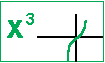## Polynomial Functions: Notes

Other pages
What's A Function?
Dilation by a constant
Dilation
Polynomial Functions
Rational Functions

This pages
Definition & Notation
Polynomial Computation
Possible Roots
Quadratic, Cubic, Quartic Formulas
Complex Roots

 Definition & Notation Simple definition:   polynomial   Precalc definition -- an algebraic expression with variable x,   anxn + an-1xn-1 + an-2xn-2 + ... +a2x2 + a1x1 + a0x0,   in which the constants an, an-1, an-2, ... , a2, a1, a0 are real, and serve as coefficients or the constant term, and in which all exponent of x are counting numbers.     The polynomial is named or identitied by the term of the highest power. constant term: a0x0 or a0 linear term: a1x1 quadratic term: a2x2 cubic term: a3x3 . quartic term: a4x4 quintic term: a5x5 Polynomials of even powers are similiar as they     "start high & end high." Polynomials of odd powers are similiar as they     "start low & end high."See highest degree for the impact on the graph and features of the polynomial.  Leading coefficient -- The coefficient of the term of highest degree. For polynomial anxn + ...,     If an is positive, the polynomial is not reflected.     If an is negative, the polynomial is reflected.See leading coefficient and the impact on the graph and features of the polynomial including overall and end behavior.

 Polynomial Computation All rules of arithmetic and algebra hold for polynomials. You may add, subtract, multiply, divide, and raise a polynomial to a power. All rules of the computation of functions hold for polynomials. Since a polynomial is a function, you may add, subtract, multiply, divide, and create compositions of polynomials. Composition (creating from other) of functions sum (f + g)(x) difference (f - g)(x) product (f · g)(x) quotient (f ÷ g)(x) composition (f o g)(x)= f(g(x))   Play with Polynomial Graphs polynomial spreadsheet -- displays polynomial graphs in linear & quadratic factor form and anxn + ... form     Division Algorithm - Use division to rewrite a function as the product of two factors plus a remainder. f(x) = (quotient function) · (divisor function) + (remainder function) If the remainder is 0, the divisor evenly divides the original function. Examples of the above. ex 1. y = x2 + 4x + 4 = (x + 2)(x + 2) + 0 ex 2. y = x2 + 4x + 5 = (x + 2)(x + 2) + 1 ex 3. y = x2 + 6x + 4 = (x + 2)(x + 2) + 2x ex 4. y = 2x2 + 4x + 4 = (x + 2)(x + 2) + x²

Possible Roots
Factor Theorem --
The polynomial (x-r) is a divisor (and factor) of the polynomial f(x) if and only if
r is a root or zero of f(x)
Examples of the above.
ex 1. (x2 + 4x + 4)/(x + 2) = (x + 2) + 0,
because x = -2 is a root of y=x2 + 4x + 4

ex 2.(x2 + 4x + 5) = (x + 2)(x + 2) + 1
because x = -2 is not a root of y=x2 + 4x + 5.
When x = -2, y=x2 + 4x + 5 is equal to 1.

Integral Zero Theorem --
The integer r is a root of the polynomial f(x) if and only if
r is a divisor of the constant term, a0.
Examples of the above.
ex 1. -2 is a root of y=x2 + 4x + 4,
and -2 is a factor of 4, the constant term.

Rational Zero Theorem --
The rational number, fraction, p/q is a root of the polynomial f(x) if and only if
p is a factor of the constant term and
q is a factor of the leading coefficient.
Examples.
 Solve: Solution(s): p's, factors ofconstant term q's, factors of leading coefficient p/q's, possible rational root 3x + 4 = 0 x = -4/3 ± 1, ±2, ±4 ±1, ±3 ± 1/1, ±2/1, ±4/1, ± 1/3, ±2/3, ±4/3 4x - 3 = 0 x = -3/4 ± 1, ±3 ±1, ±2, ±4 ± 1/1, ±3/1, ± 1/2, ±3/2, ± 1/4, ±3/4 x + 3 = 0 x = -3 ± 1, ±3 ±1 ± 1/1, ±3/1 (x + 2)(x - 5) = 0   x2 - 3x - 10 = 0 x = -2, x= 5 ± 1, ±2, ±5 ±1 ± 1, ±2, ±5 x3 + 5x2 + 2x - 8 = 0 x = ? ± 1, ±2, ± 4, ±8 ±1 ± 1, ±2, ± 4, ±8

Irrational Zeros --
Use the quadratic formula for 2nd degree polynomials.
Use the The "Cubic Formula" by Helmut Knaust at http://www.sosmath.com/algebra/factor/fac11/fac11.html for 3rd degree polynomials.
Use the "Quartic Formula" by planetmath.org at http://planetmath.org/encyclopedia/QuarticFormula.html for 4th degree polynomials.
There DO NOT EXIST formulas for 5th degree or higher polynomials.
Approximate roots by determining sign changes in successive values of the function.

Complex Roots
Complex roots come in pairs.
If a + bi is a root, then its conjugate, a - bi is a root.
Seek these using the quadratic formula.

## MATHEMATICAL CONCEPTS, inc.

85 First Street, Keyport, NJ 07735-1503
callus@mathnstuff.com     (732) 739-3951
© 2/22/2004, www.mathnstuff.com/math/spoken/here/2class/300/fx/polyn.htm